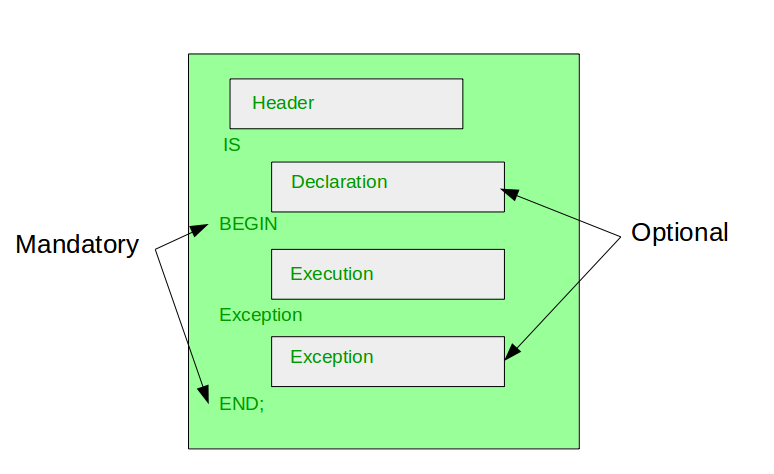# Blocks in PL/SQL

In PL/SQL, All statements are classified into units that is called Blocks. PL/SQL blocks can include variables, SQL statements, loops, constants, conditional statements and exception handling. Blocks can also build a function or a procedure or a package.Broadly, PL/SQL blocks are two types: Anonymous blocks and

1. Anonymous blocks: In PL/SQL, That’s blocks which is not have header are known as anonymous blocks. These blocks do not form the body of a function or triggers or procedure.

Example: Here a code example of find greatest number with Anonymous blocks.

 `DECLARE`  `    ``-- declare variable a, b and c  ` `    ``-- and these three variables datatype are integer   ` `   ``a number;  ` `   ``b number;  ` `   ``c number;  ` `BEGIN`  `   ``a:= 10;  ` `   ``b:= 100;  ` `    ``--find largest number ` `    ``--take it in c variable ` `   ``IF a > b ``THEN`  `      ``c:= a;  ` `   ``ELSE`  `      ``c:= b;  ` `   ``END` `IF; ` `   ``dbms_output.put_line(``' Maximum number in 10 and 100: '` `|| c);  ` `END``;  ` `/  ` `-- Program End  `

Output:

```Maximum number in 10 and 100: 100
```

2. Named blocks: That’s PL/SQL blocks which having header or labels are known as Named blocks. These blocks can either be subprograms like functions, procedures, packages or Triggers.

Example: Here a code example of find greatest number with Named blocks means using function.

 `DECLARE`  ` `  `    ``-- declare variable a, b and c  ` `    ``-- and these three variables datatype are integer   ` `DECLARE`  `   ``a number;  ` `   ``b number;  ` `   ``c number;  ` `   ``--Function return largest number of   ` `   ``-- two given number ` `FUNCTION` `findMax(x ``IN` `number, y ``IN` `number)   ` `RETURN` `number  ` `IS`  `    ``z number;  ` `BEGIN`  `   ``IF x > y ``THEN`  `      ``z:= x;  ` `   ``ELSE`  `      ``Z:= y;  ` `   ``END` `IF;   ` `   ``RETURN` `z;  ` `END``;  ` `BEGIN`  `   ``a:= 10;  ` `   ``b:= 100;   ` `   ``c := findMax(a, b);  ` `   ``dbms_output.put_line(``' Maximum number in 10 and 100 is: '` `|| c);  ` `END``;  ` `/  ` `-- Program End  `

Output:

```Maximum number in 10 and 100: 100
```

My Personal Notes arrow_drop_upStrategy Path planning and Destination matters in success No need to worry about in between temporary failures

If you like GeeksforGeeks and would like to contribute, you can also write an article using contribute.geeksforgeeks.org or mail your article to contribute@geeksforgeeks.org. See your article appearing on the GeeksforGeeks main page and help other Geeks.

Please Improve this article if you find anything incorrect by clicking on the "Improve Article" button below.

Article Tags :
Practice Tags :

2

Please write to us at contribute@geeksforgeeks.org to report any issue with the above content.